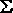### Digital Logic Circuits - QUINE-McCLUSKEY MINIMIZATION Method

QUINE-McCLUSKEY MINIMIZATION

Quine-McCluskey minimization method uses the same theorem to produce the solution as the K-map method, namely X(Y+Y')=X

Minimization Technique

• The expression is represented in the canonical SOP form if not already in that form.
• The function is converted into numeric notation.
• The numbers are converted into binary form.
• The minterms are arranged in a column divided into groups.
• Begin with the minimization procedure.
• Each minterm of one group is compared with each minterm in the group immediately below.
• Each time a number is found in one group which is the same as a number in the group below except for one digit, the numbers pair is ticked and a new composite is created.
• This composite number has the same number of digits as the numbers in the pair except the digit different which is replaced by an "x".
• The above procedure is repeated on the second column to generate a third column.
• The next step is to identify the essential prime implicants, which can be done using a prime implicant chart.
• Where a prime implicant covers a minterm, the intersection of the corresponding row and column is marked with a cross.
• Those columns with only one cross identify the essential prime implicants. -> These prime implicants must be in the final answer.
• The single crosses on a column are circled and all the crosses on the same row are also circled, indicating that these crosses are covered by the prime implicants selected.
• Once one cross on a column is circled, all the crosses on that column can be circled since the minterm is now covered.
• If any non-essential prime implicant has all its crosses circled, the prime implicant is redundant and need not be considered further.
• Next, a selection must be made from the remaining nonessential prime implicants, by considering how the non-circled crosses can be covered best.
• One generally would take those prime implicants which cover the greatest number of crosses on their row.
• If all the crosses in one row also occur on another row which includes further crosses, then the latter is said to dominate the former and can be selected.
• The dominated prime implicant can then be deleted.

Example

Find the minimal sum of products for the Boolean expression,

f=(1,2,3,7,8,9,10,11,14,15), using Quine-McCluskey method.

Firstly these minterms are represented in the binary form as shown in the table below. The above binary representations are grouped into a number of sections in terms of the number of 1's as shown in the table below.

Binary representation of minterms

 Minterms U V W X 1 0 0 0 1 2 0 0 1 0 3 0 0 1 1 7 0 1 1 1 8 1 0 0 0 9 1 0 0 1 10 1 0 1 0 11 1 0 1 1 14 1 1 1 0 15 1 1 1 1

Group of minterms for different number of 1's

 No of 1's Minterms U V W X 1 1 0 0 0 1 1 2 0 0 1 0 1 8 1 0 0 0 2 3 0 0 1 1 2 9 1 0 0 1 2 10 1 0 1 0 3 7 0 1 1 1 3 11 1 0 1 1 3 14 1 1 1 0 4 15 1 1 1 1

Any two numbers in these groups which differ from each other by only one variable can be chosen and combined, to get 2-cell combination, as shown in the table below.

2-Cell combinations

 Combinations U V W X (1,3) 0 0 - 1 (1,9) - 0 0 1 (2,3) 0 0 1 - (2,10) - 0 1 0 (8,9) 1 0 0 - (8,10) 1 0 - 0 (3,7) 0 - 1 1 (3,11) - 0 1 1 (9,11) 1 0 - 1 (10,11) 1 0 1 - (10,14) 1 - 1 0 (7,15) - 1 1 1 (11,15) 1 - 1 1 (14,15) 1 1 1 -

From the 2-cell combinations, one variable and dash in the same position can be combined to form 4-cell combinations as shown in the figure below.

 Combinations U V W X (1,3,9,11) - 0 - 1 (2,3,10,11) - 0 1 - (8,9,10,11) 1 0 - - (3,7,11,15) - - 1 1 (10,11,14,15) 1 - 1 -

The cells (1,3) and (9,11) form the same 4-cell combination as the cells (1,9) and (3,11). The order in which the cells are placed in a combination does not have any effect. Thus the (1,3,9,11) combination could be written as (1,9,3,11).

From above 4-cell combination table, the prime implicants table can be plotted as shown in table below.

Prime Implicants Table

 Prime Implicants 1 2 3 7 8 9 10 11 14 15 (1,3,9,11) X - X - - X - X - - (2,3,10,11) - X X - - - X X - - (8,9,10,11) - - - - X X X X - - (3,7,11,15) - - - - - - X X X X - X X - X X - - - X -

The columns having only one cross mark correspond to essential prime implicants. A yellow cross is used against every essential prime implicant. The prime implicants sum gives the function in its minimal SOP form.

Y = V'X + V'W + UV' + WX + UW